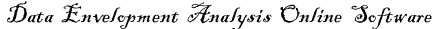# Returns to scale

Returns to scale refers to a technical property of production that examines changes in output subsequent to a proportional change in all inputs (where all inputs increase by a constant). If output increases by that same proportional change then there are constant returns to scale (CRTS), sometimes referred to simply as returns to scale. If output increases by less than that proportional change, there are decreasing returns to scale (DRS). If output increases by more than that proportion, there are increasing returns to scale (IRS)...

Short example: Where all inputs increase by a factor of 2, new values for output should be: